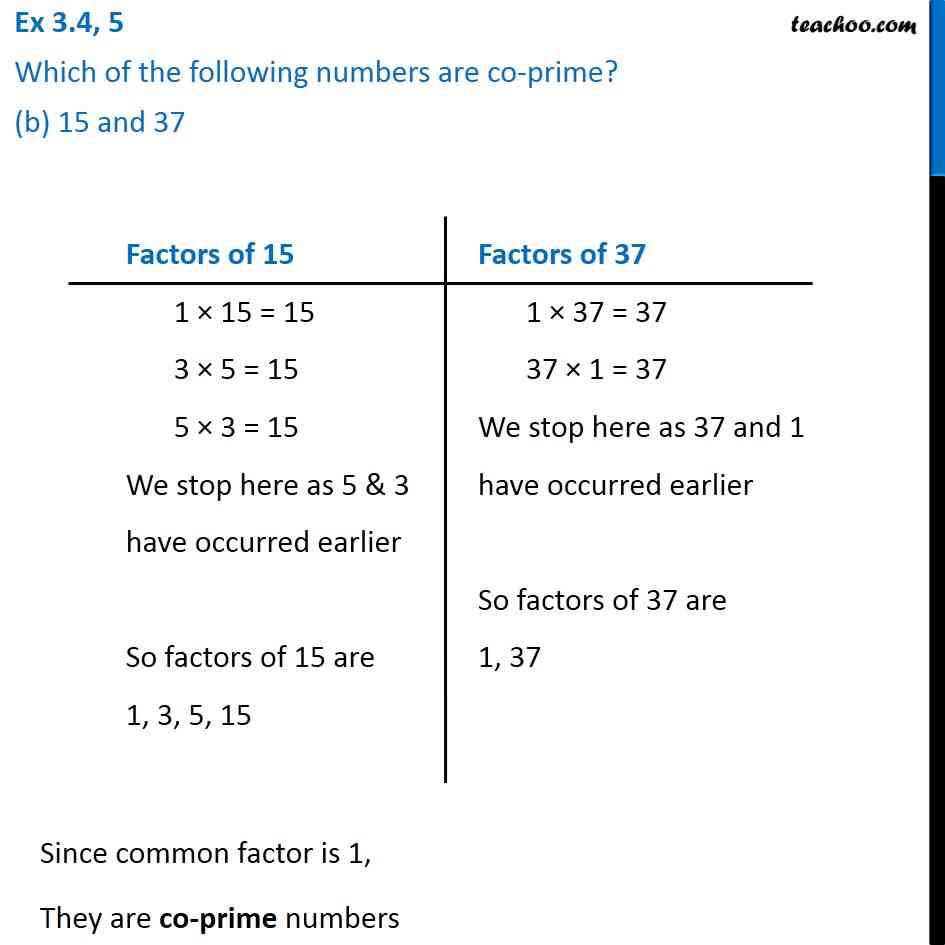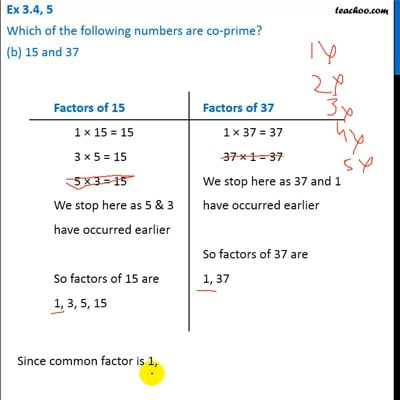Ex 3.4

Chapter 3 Class 6 Playing with Numbers
Serial order wiseThis video is only available for Teachoo black users

Introducing your new favourite teacher - Teachoo Black, at only ₹83 per month

### Transcript

Ex 3.4, 5 Which of the following numbers are co-prime? (b) 15 and 37 Factors of 15 1 × 15 = 15 3 × 5 = 15 5 × 3 = 15 We stop here as 5 & 3 have occurred earlier So factors of 15 are 1, 3, 5, 15 Factors of 37 1 × 37 = 37 37 × 1 = 37 We stop here as 37 and 1 have occurred earlier So factors of 37 are 1, 37 Since common factor is 1, They are co-prime numbers